# Create Level of Detail, Rank, and Tile Calculations

Note: Starting in version 2020.4.1, you can now create and edit flows in Tableau Server and Tableau Cloud. The content in this topic applies to all platforms, unless specifically noted. For more information about authoring flows on the web, see Tableau Prep on the Web in the Tableau Server(Link opens in a new window) and Tableau Cloud(Link opens in a new window) help.

You can use calculated fields to create new data using data that already exists in your data source. Tableau Prep supports many of the same calculation types as Tableau Desktop. For general information about creating calculations, see Get Started with Calculations in Tableau(Link opens in a new window).

Starting in version 2020.1.3 Tableau Prep Builder and on the web, you can use FIXED Level of Detail (LOD) and RANK and ROW_NUMBER analytic functions to perform more complex calculations.

For example, add a FIXED LOD calculation to change the granularity of fields in your table, use the ROW_NUMBER () analytic function to quickly find duplicate rows, or use one of the RANK () functions to find the top N or bottom N values for a selection of rows with similar data. If you want a more guided experience when building these types of expressions, you can use the visual calculation editor.

Starting in version 2021.4.1 Tableau Prep Builder and on the web, you can use the tile feature to distribute rows into a specified number of buckets.

Note: Some functions supported in Tableau Desktop may not yet be supported in Tableau Prep. To view the available functions for Tableau Prep, review the function list in the Calculation editor.

## Calculate level of detail

When you need to calculate data at multiple levels of granularity in the same table, you can write a level of Detail (LOD) expression to do this. For example, if you wanted to find the total sales for each region, you could write a calculation like `{FIXED [Region] : SUM([Sales])}`.

Tableau Prep supports the FIXED level of detail expression and uses the syntax `{FIXED [Field1],[Field2] : Aggregation([Field)}`.

LOD expressions have two parts to the equation that are separated by a colon.

• FIXED [Field] (required): This is the field or fields that you want to calculate the values for. For example if you wanted to find the total sales for customer and region, you would enter `FIXED [Customer ID], [Region]:`. If you don't select any fields, this is the equivalent to performing the aggregation defined on the right side of the colon and repeating that value for every row.

• Aggregation ([Field]) (required): Select what you want to calculate and what level of aggregation you want. For example if you want to find the total sales, then enter `SUM([Sales]`.

When using this feature in Tableau Prep, the following requirements apply:

• `INCLUDE` and `EXCLUDE` LOD expressions aren't supported.
• Aggregation calculations are only supported inside an LOD expression. For example, `SUM([Sales])` would not be valid, but `{FIXED [Region] : SUM([Sales])}` is valid.
• Nesting expressions inside an LOD expression isn't supported. For example, `{ FIXED [Region] : AVG( [Sales] ) / SUM( [Profit] )}` isn't supported.

• Combining an LOD expression with another expression isn't supported.  For example `[Sales]/{ FIXED [Country / Region]:SUM([Sales])}` isn't supported.
• ### Create Level of Detail (LOD) calculations

To create a level of detail calculation, you can use the Calculation editor to write the calculation yourself or if you want a more guided experience, you can use the Visual Calculation editor where you select your fields and Tableau Prep writes the calculation for you.

#### Calculation editor

1. In the Profile pane toolbar click Create Calculated Field, or in a profile card or data grid, click the More options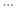menu and select Create Calculated Field > Custom Calculation.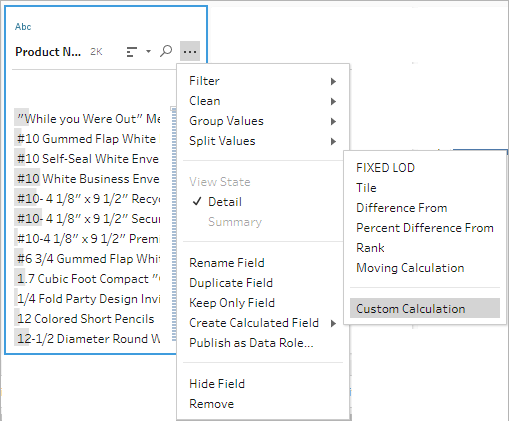2. In the Calculation editor, enter a name for your calculation and enter the expression.

For example, to find the average days to ship products by city, create a calculation like the one shown below.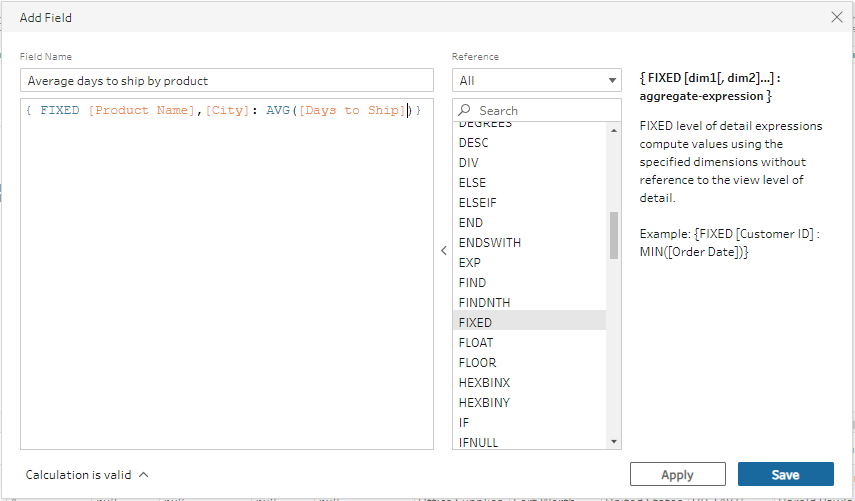#### Visual calculation editor

Select fields from a list and Tableau Prep builds the calculation for you as you make your selections. A preview of the results is shown in the left pane so you can see the results of your selections as you go.

1. In a profile card or results pane, click the More optionsmenu and select Create Calculated Field >Fixed LOD.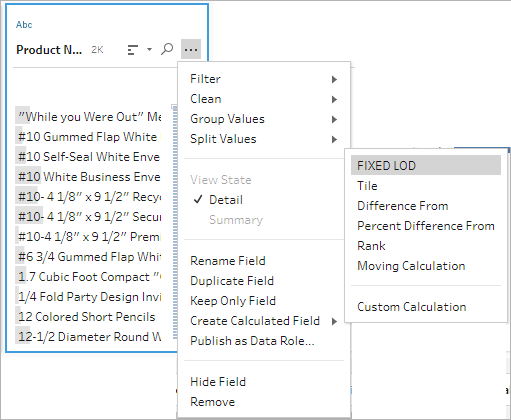2. In the Visual Calculation editor, do the following:

• In the Group by section, select the fields that you want to calculate the values for. The field where you selected the Create Calculated Field >Fixed LOD menu option is added by default. Click the plus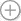icon to add any additional fields to your calculation. This populates the left side of the equation, `{FIXED [Field1],[Field2] :`.
• In the Compute using section, select the field that you want to use to calculate your new values. Then select your aggregation. This populates the right side of the equation, `Aggregation([Field)}`.

A graphic below the field shows the distribution of values and a total count for each value combination. Depending on the type of data, this can be a box plot, range of values, or the actual values.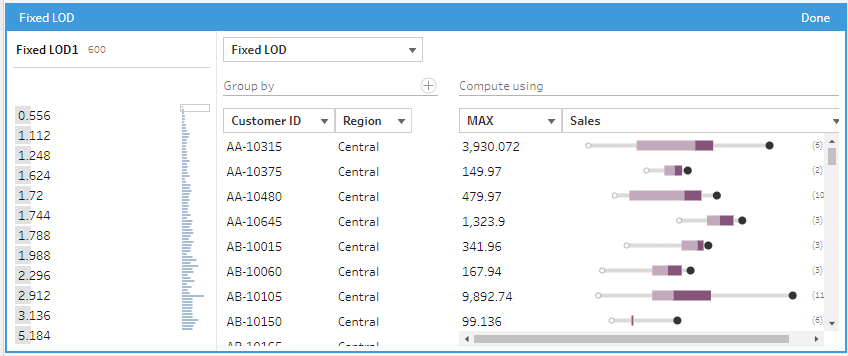Note: Available aggregation values vary by the data type assigned to the field.

• To remove a field, right-click or Cntrl-click (MacOS) in the drop-down box for the fields in the Group by section and select Remove Field.

• In the left pane, double-click in the field header and enter a name for your calculation.
3. Click Done to add your new calculated field. In the Changes pane, you can see the calculation that Tableau Prep generated. Click Edit to open the visual calculation editor to make any changes.## Calculate rank or row number

Analytic functions, sometimes referred to as window calculations, enable you to perform calculations across the entire table, or a selection of rows (partition) in your data set. For example, when applying a rank to a selection of rows, you would use the following calculation syntax:

`{PARTITION [field]: {ORDERBY [field]: RANK() }}`

• PARTITION (optional): Designate the rows you want to perform the calculation on. You can specify more than one field, but if you want to use the entire table, omit this part of the function and Tableau Prep treats all the rows as the partition. For example `{ORDERBY [Sales] : RANK() }`.

• ORDERBY (required): Specify one or more fields that you want to use to generate the sequence for the rank.

• Rank () (required): Specify the rank type or ROW_NUMBER () you want to calculate. Tableau Prep supports RANK(), RANK_DENSE(), RANK_MODIFIED(), RANK_PERCENTILE(), and ROW_NUMBER() functions.

• DESC or ASC (optional): Represents descending (DESC) or ascending (ASC) order. By default, rank is sorted in descending order, so you don't need to specify this in the expression. If you want to change the sort order, add ASC to the expression.

You can also include both options in the function. For example if you wanted to rank a selection of rows, but wanted to sort the rows in ascending order, then apply the rank in descending order, you would include these two options in the expression. For example: `{PARTITION [Country], [State]: {ORDERBY [Sales] ASC,[Customer Name] DESC: RANK() }}`

When using this feature, the following requirements apply:

• Nesting expressions inside a RANK () function isn't supported.  For example, `[Sales]/{PARTITION [Country]: {ORDERBY [Sales]: RANK() }} / SUM( [Profit] )}` isn't supported.
• Combining a RANK () function with another expression isn't supported.  For example `[Sales]/{PARTITION [Country]: {ORDERBY [Sales]: RANK() }}` isn't supported.

### Supported analytic functions

Function Description Result
RANK ()

Assigns a whole number rank starting with 1, in ascending or descending order to each row. If rows have the same value, they share the rank that is assigned to the first instance of the value. The number of rows with the same rank is added when calculating the rank for the next row, so you may not get consecutive rank values.

Sample calculation:`{ORDERBY [Commission] DESC: RANK()}`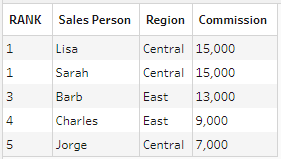RANK_DENSE()

Assigns a whole number rank starting with 1 in ascending or descending order to each row. If rows have the same value, they share the rank that is assigned to the first instance of the value, but no rank values are skipped so you will see consecutive rank values.

Sample calculation:`{ORDERBY [Commission] DESC: RANK_DENSE()}`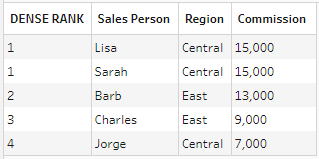RANK_MODIFIED()

Assigns a whole number rank starting with 1, in ascending or descending order to each row. If rows have the same value, they share the rank that is assigned to the last instance of the value. Rank_Modified is calculated as `Rank + (Rank + Number of duplicate rows - 1)`.

Sample calculation:`{ORDERBY [Commission] DESC: RANK_MODIFIED()}`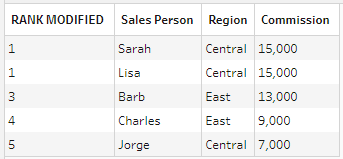RANK_PERCENTILE()

Assigns a percentile rank from 0 to 1 in ascending or descending order to each row. RANK_PERCENTILE is calculated as `(Rank-1)/(Total rows-1)`.

Sample calculation:`{ORDERBY [Commission] DESC: RANK_PERCENTILE()}`

Note: In the event of a tie, Tableau Prep rounds the rank down, similar to PERCENT_RANK() in SQL.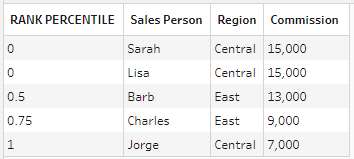ROW_NUMBER()

Assigns a sequential row ID to each unique row. No row number values are skipped. If you have duplicate rows and use this calculation, your results might change each time you run the flow if the order of rows changes.

Sample calculation:`{ORDERBY [Commission] DESC: ROW_NUMBER()}`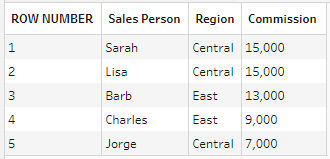The following example shows a comparison of each of the above functions applied to the same data set.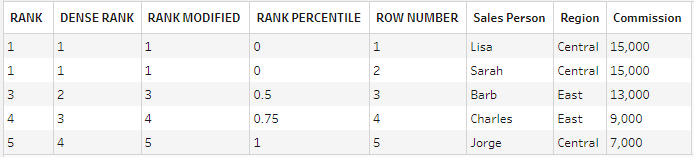### Create Rank or Row Number calculations

To create a Rank or Row_Number calculations, you can use the Calculation editor to write the calculation yourself or if you want a more guided experience, you can use the Visual Calculation editor where you select your fields and Tableau Prep writes the calculation for you.

Note: ROW_NUMBER () calculations aren't available in the visual calculation editor.

#### Calculation editor

Use the Calculation editor to create any of the supported RANK () or ROW_NUMBER() calculations. The list of supported analytic calculations is shown in the Calculation editor in the Reference drop-down under Analytic.

1. In the Profile pane toolbar click Create Calculated Field, or in a profile card or data grid, click the More optionsmenu and select Create Calculated Field > Custom Calculation.2. In the Calculation editor, enter a name for your calculation and enter the expression.

For example to find the latest customer order, create a calculation like the one shown below, then keep only the customer order rows that are ranked with the number 1.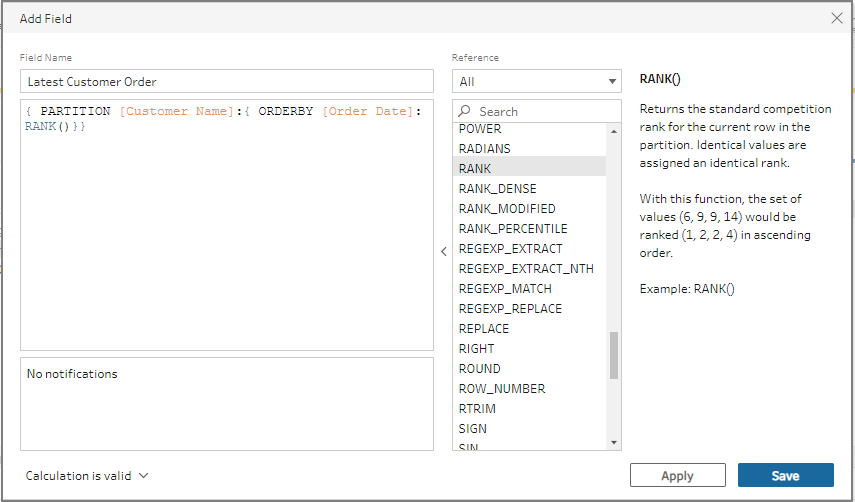Example: Use ROW_NUMBER to find and remove duplicate values.

This example uses the Superstore sample data set in Tableau Prep Builder to find and remove exact duplicate values for the field Row ID using the ROW_NUMBER function.

1. Open the Sample Superstore flow.

2. In the Flow pane, for the Input step Orders West, click on the Clean step Rename States.

3. In the toolbar, click Create Calculated Field.

4. In the Calculation editor, name the new field "Duplicates", and use the `ROW_NUMBER` function to add a row number to the field Row ID using the expression `{PARTITION [Row ID]: {ORDERBY[Row ID]:ROW_NUMBER()}}` and click Save.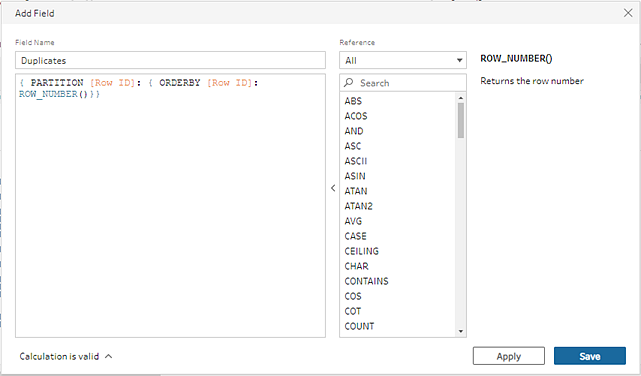5. In the new calculated field, right-click or Cmd-click (MacOS) on the field value 1, then select Keep Only from the menu.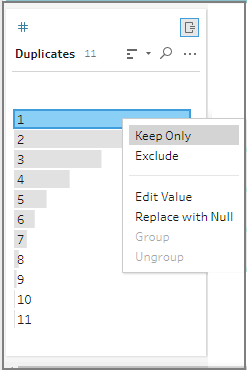Before After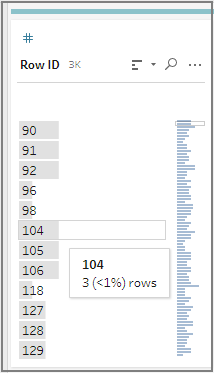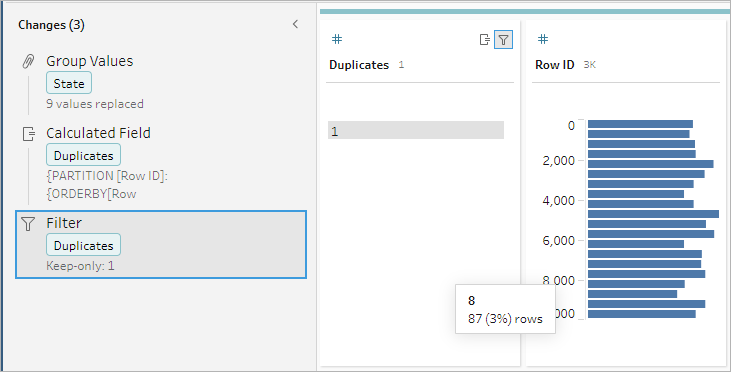#### Visual Calculation editor

Just like when creating a level of detail calculation, you can use the visual calculation editor to build a rank calculation. Select the fields you want to include in the calculation, then select the fields you want to use to rank the rows and the type of rank you want to calculate. A preview of the results is shown in the left pane so you can see the results of your selections as you go.

1. In a profile card or results pane, click the More optionsmenu and select Create Calculated Field >Rank.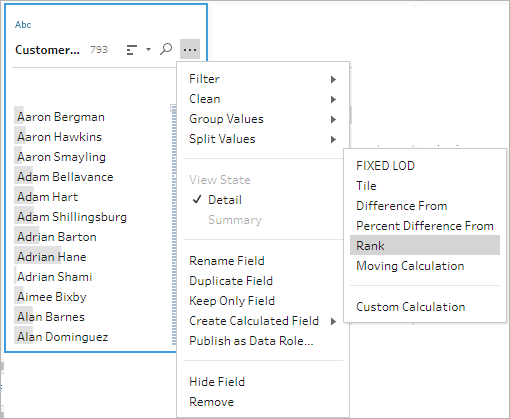2. In the Visual calculation editor, do the following:

• In the Group by section, select the fields with rows you want to compute values for. This creates the Partition part of the calculation.

After you select your first field, click the plusicon to add any additional fields to your calculation. If you want to include all rows or remove a selected field, right-click or Cmd-click (MacOS) in the drop-down box for the fields in the Group by section and select Remove Field.

• In the Order by section, select the fields that you want to use to rank your new values. The field where you selected the Create Calculated Field >Rank menu option is added by default.

Click the plusicon to add any additional fields to your calculation, then select your Rank type. Click the sort icon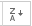to change the rank order from descending (DESC) to ascending (ASC).

Note: Rank values vary by the data type assigned to the field.

• In the left pane, double-click in the field header and enter a name for your calculation.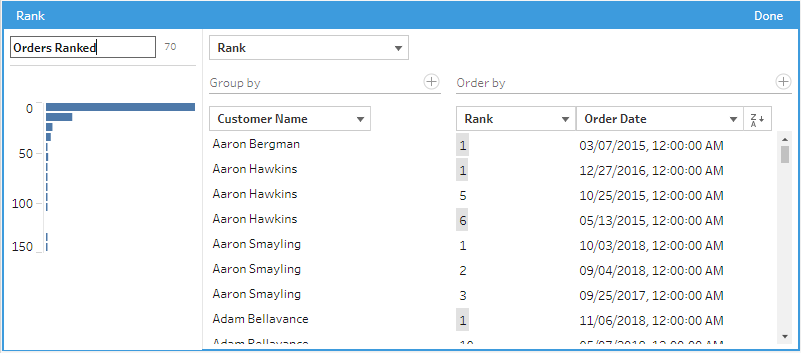3. Click Done to add your new calculated field. In the Changes pane, you can see the calculation that Tableau Prep Builder generated. Click Edit to open the visual calculation editor to make any changes.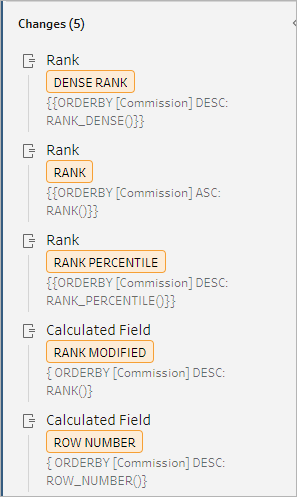## Calculate tiles

Use the Tile feature to distribute rows into a specified number of buckets by creating a calculated field. You select the fields that you want to distribute by, and the number of groups (tiles) to be used. You can also select additional fields for creating partitions where the tiled rows are distributed into groups. Use the Calculation editor to input the syntax manually or use the Visual Calculation editor to select the fields and Tableau Prep writes the calculation for you.

For example, if you have rows of student data and wanted to see which students are in the top 50% and bottom 50%, you can group the data into two tiles.The following example shows two groups for the upper and lower half of student grades. The syntax for this method is:

`{ORDERBY [Grade] DESC:NTILE(2)}`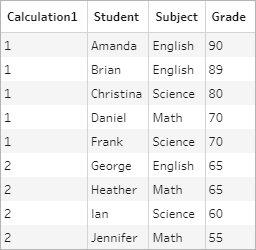You can also create a partition, where each value of a field is a separate partition, and divide data into groups for each partition.

The following example shows creating partitions for the Subject field. A partition is created for each subject and two groups (tiles) are created for the Grade field. The rows are then distributed evenly into the two groups for the three partitions. The syntax for this method is:

`{PARTITION [Subject]:{ORDERBY [Grade] DESC:NTILE(2)}}`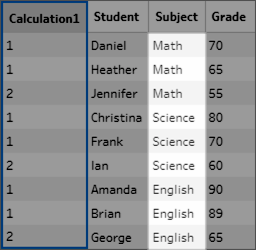### Create Tile calculations

To create tile calculations, you can use the Calculation editor to write the calculation yourself or if you want a more guided experience, you can use the Visual Calculation editor where you select your fields and Tableau Prep writes the calculation for you.

#### Visual Calculation editor

When you use the visual calculation editor to create a tile calculation, a preview of the results is shown in the left pane.

1. Select a profile card to create a tile calculation.

2. Click the More options menu and select Create Calculated Field > Tile.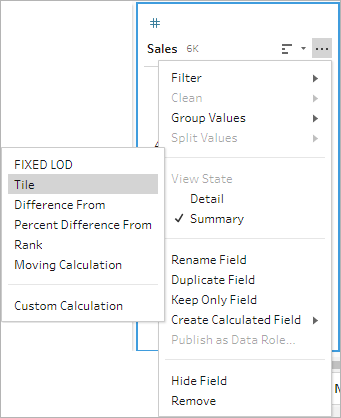The selected profile card is added as an ORDERBY field.

3. In the Visual calculation editor, do the following:

• Select the number of tile groupings you want. The default value for Tiles is 1.

• In the Group by section, select the fields for the rows you want to compute values for. This creates the `PARTITION` part of the calculation. You can have multiple Group by fields for a single calculation.

Click the plusicon to add any additional fields to your calculation. If you want to include all rows or remove a selected field, right-click or Cmd-click (MacOS) in the drop-down box for the fields in the Group by section and select Remove Field.

• In the left pane, double-click in the field header and enter a name for your calculation.

• In the Order by section, select one or more fields that you want to use to group and distribute your new values. You must have at least one Order by field. The field where you selected the Create Calculated Field >Tile menu option is added by default.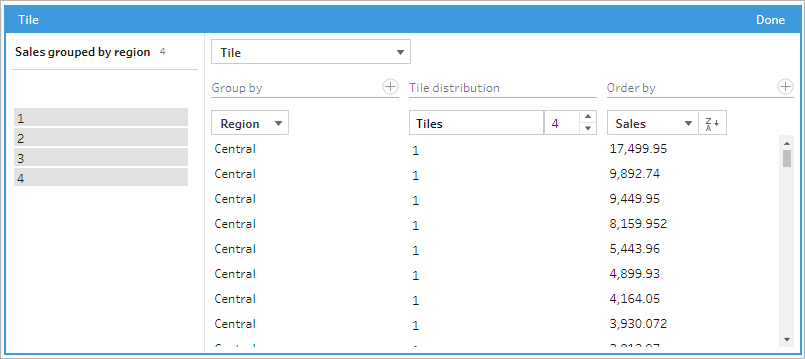4. To sort the results, do the following:

• Click any of the Calculation rows to filter the results for the selected grouping

• Change the ascending or descending order of the order by field.

6. In the Changes pane, you can see the calculation that Tableau Prep Builder generated. Click Edit to open the visual calculation editor to make any changes.

The following example shows a quartile division of rows. A partition is created based on four US regions and then the Sales field data is evenly grouped into the partitions.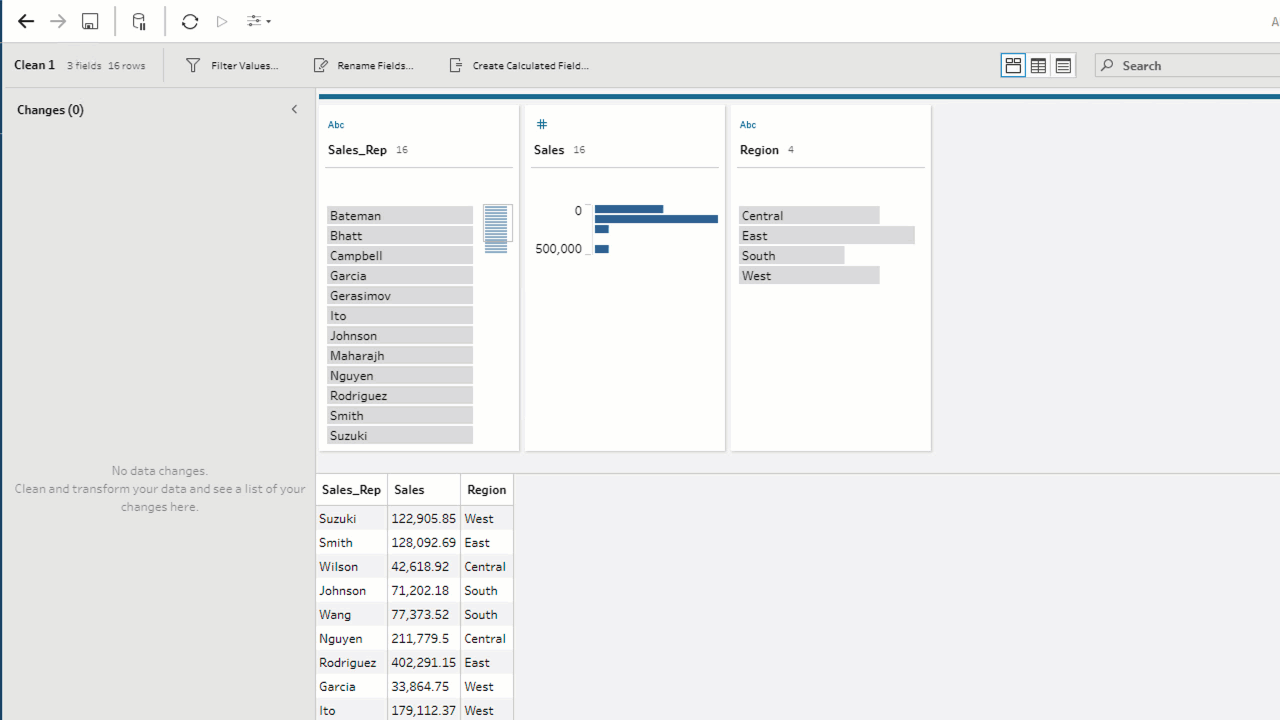#### Calculation editor

1. In the Profile pane toolbar, click Create Calculated Field, or in a profile card or data grid, click the More optionsmenu and select Create Calculated Field > Custom Calculation.2. In the Calculation editor, enter a name for your calculation and enter the expression. For example, to order rows of students by grades into two groups and then group them by subject, use : `{PARTITION [Subject]:{ORDERBY [Grade] DESC:NTILE(2)}}`.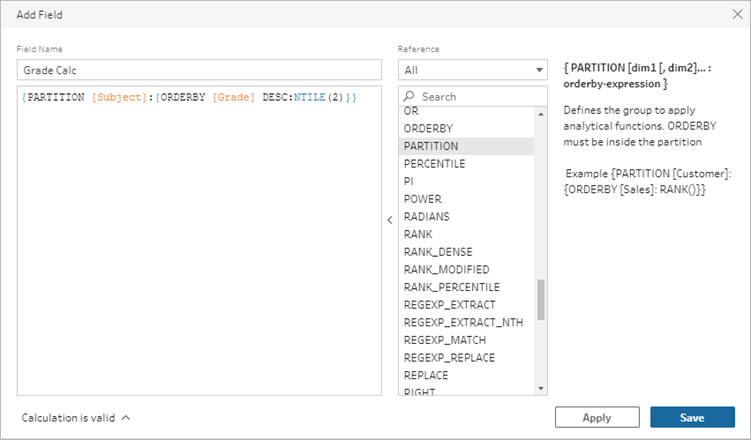Tile calculations include the following elements:

• PARTITION (optional): A partition clause differs the rows of a result set into partitions where the NTILE() function is used.

• ORDERBY (required) The ORDER BY clause defines the distribution of rows in each partition where the NTILE() is used.

• NTILE (required):NTILE is the integer into which the rows are divided.

Note: When all of the rows are divisible by the NTILE clause, the feature divides the rows evenly among the number of tiles. When the number of rows isn’t divisible by the NTILE clause, the resulting groups are divided into different sized bins.

• DESC or ASC (optional): Represents descending (DESC) or ascending (ASC) order. By default, the tile is sorted in descending order, so you don't need to specify this in the expression. If you want to change the sort order, add ASC to the expression.

3. Click Save.

The generated field shows the tile grouping (bin) assignments associated with each row in the table.